Copyright © Michael Richmond. This work is licensed under a Creative Commons License.

# Astronomical spectra

A spectrograph takes light from a source and separates it by wavelength, so that the red light goes in one direction, the yellow light in another direction, the blue light in another direction, and so forth. There are two basic ways of dispersing light. You can pass it through a prism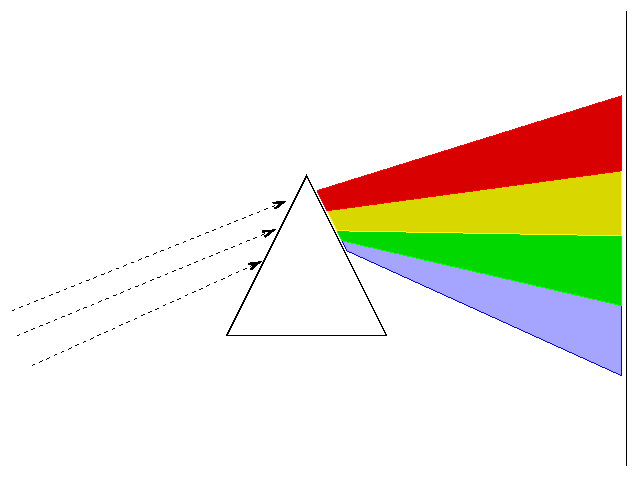or you can bounce it off (or pass it through) a diffraction grating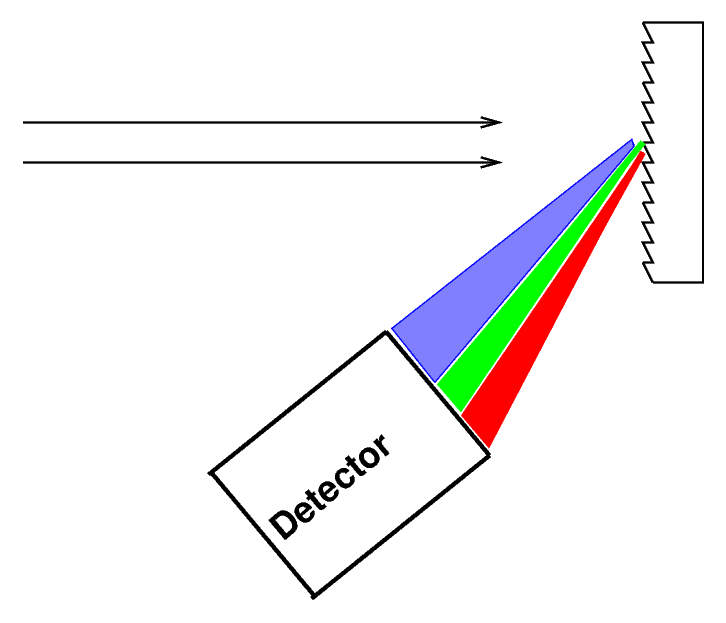```

Q:  Most astronomers these days use gratings,
not prisms.  Can you guess why?

```

You can read about the physics of diffraction gratings if you want to understand exactly how and why they work.

Astronomers often place a slit over the focal plane of the telescope, centered on the object of interest.Only light which passes through this slit will strike the grating (or prism), giving the spectrum a characteristic shape: vertical lines on a long, horizontal canvas.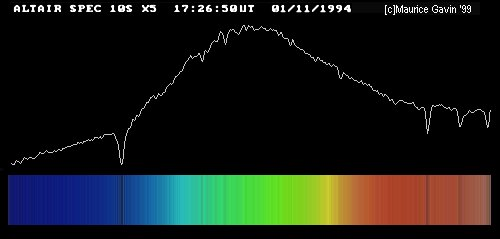Image by Maurice Gavin, from the wpo-amateur spectroscopy web site.

#### The basic types of spectrum

When we pass light from a source through a spectrgraph, we usually see one of three basic types of spectrum, depending on the nature of the source. German astronomer Gustav Kirchoff, working in the 1850s, figured out the reason for these different types of spectra. He explained the three basic types of spectra as coming from three different situations:

1. Solids, liquids, and dense gases emit light of all wavelengths, without any gaps. We call this a continuous spectrum.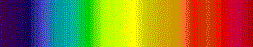2. Thin gases emit light of only a few wavelengths. We call this an emission or bright line spectrum.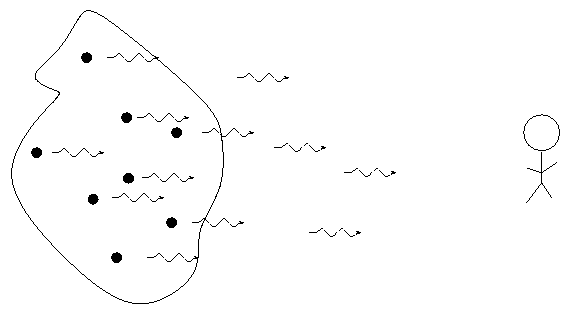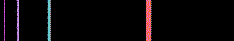3. If there is a source of light behind it, a thin gas will absorb light of the same wavelengths it emits. We call this an absorption or dark line spectrum.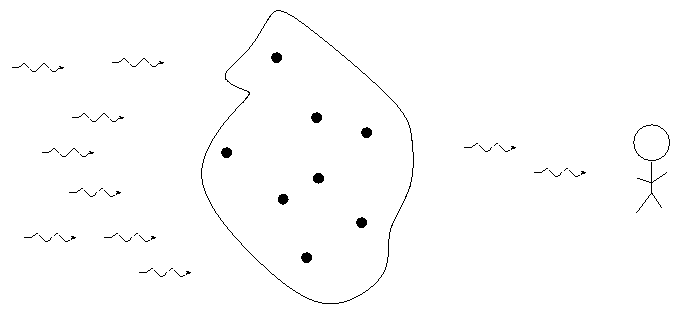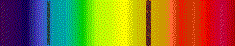#### Using spectra to identify elements

Each element generates its own unique set of wavelengths of emission or absorption.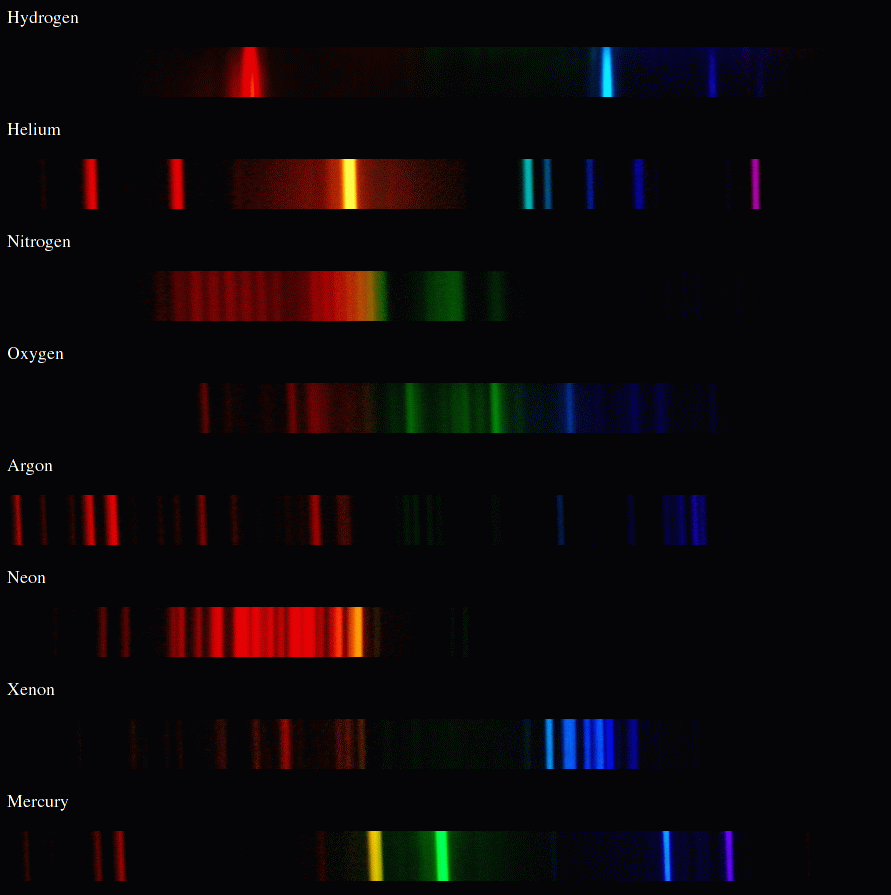We can use these patterns like fingerprints to identify the material which is emitting or absorbing light.

#### Spectra of stars

Let's consider stars, the main sources of light in the night sky. Fusion reactions at the center of a star create photons with very high energies -- gamma rays. As those photons fight their way out from the center of the star to the outer layers, they are degraded down to the visible range. So, near the outer layers of a star, light of all visible wavelengths is flying out towards the empty reaches of space.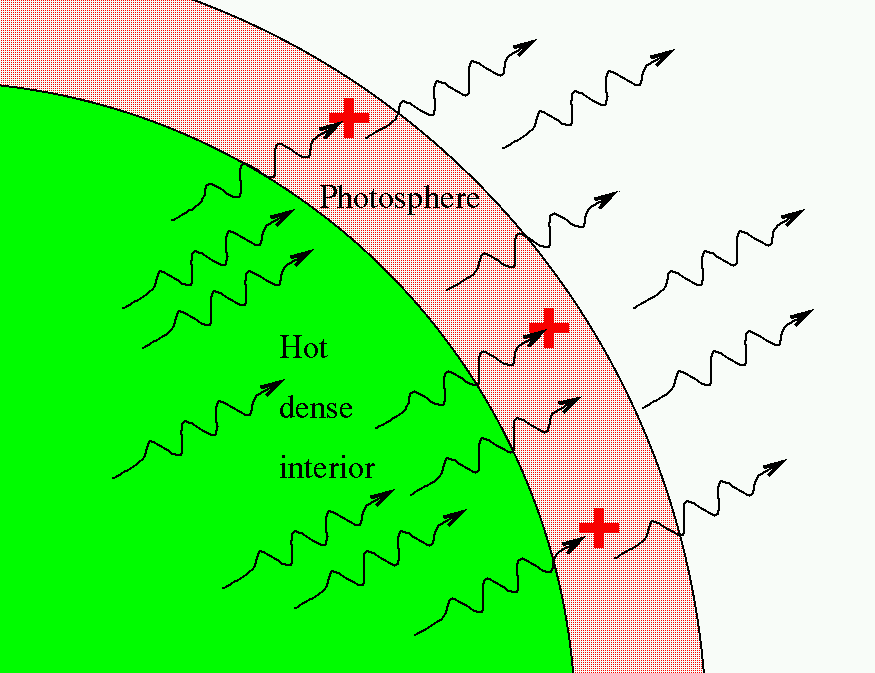However, as the photons move through one of the outermost regions of the star, called the photosphere, some of them are absorbed by atoms in that layer. The result is a spectrum which features a bright, continuous rainbow of color ... but with a few missing wavelengths.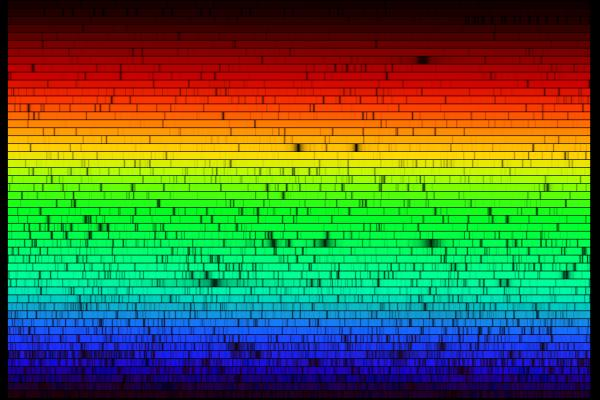If we can measure the wavelengths at which light is missing, we can identify the atoms (or ions) which are responsible for the absorption. Astronomers have spent years measuring these lines in all types of stars, and collaborated with physicists and chemists to measure the lines absorbed by known elements in the lab. In this way, astronomers can often figure out which chemical elements are inside the outer layers of a star.

#### The relativistic Doppler shift ... for small speeds

Recall the formula for the relativistic Doppler shift: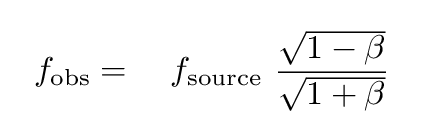We can write this in terms of the wavelength of light, instead of the frequency: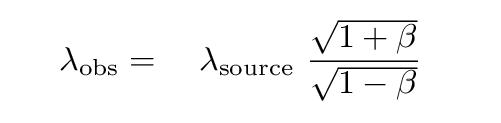If the relative speed of source and observer is much less than the speed of light, then β = v/c << 1 . In that case, we can use the binomial expansion to write an approximate value for the observed wavelength.```

Q:  Can you write a simple relationship
which is valid only at small speeds
between the ratio of wavelengths,
and the quantity v/c ?

```

Let's try using the Doppler shift to find the motion of a real star relative to the Sun. The star Arcturus shines brightly in the spring-time skies -- you can find it by following the handle of the Big Dipper.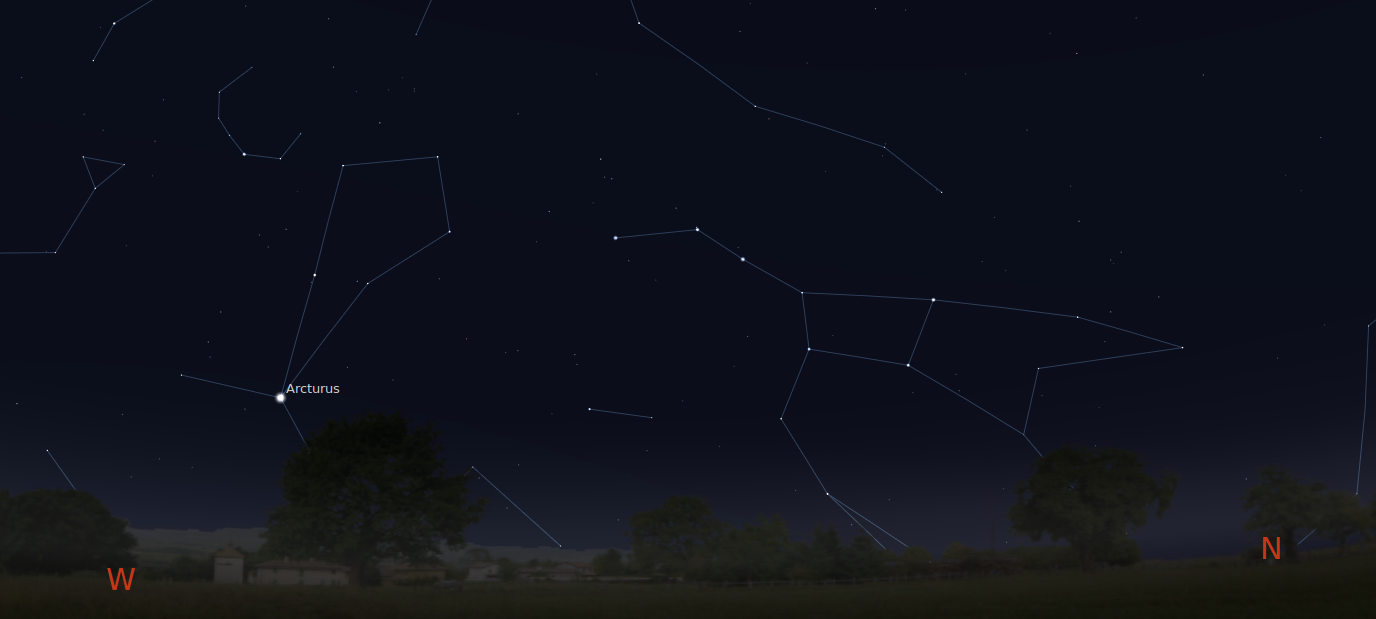If we pass the light of Arcturus through a spectrograph and record its spectrum, then compare that to the spectrum of the Sun, we see something like this: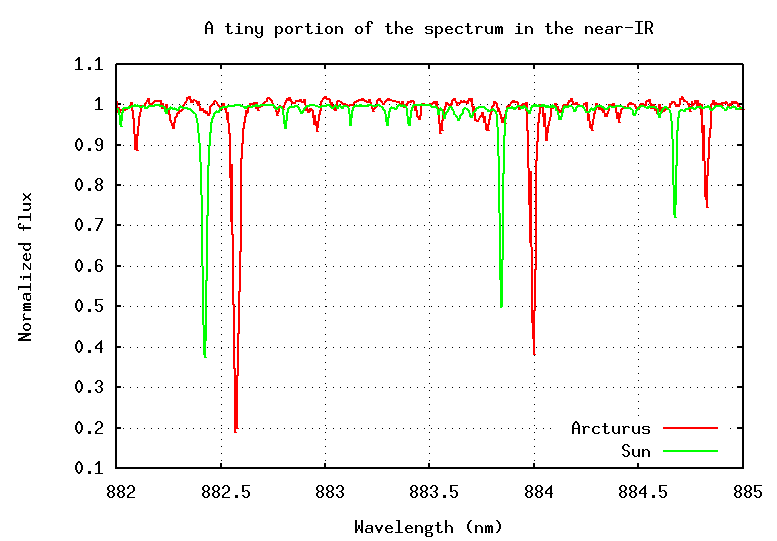```

Q:  What is the radial velocity of Arcturus?
Is it coming towards us, or going away from us?

(Half the class use the low-velocity approximation,
the other half use the full relativistic formula)

```Copyright © Michael Richmond. This work is licensed under a Creative Commons License.